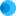Intuit HelpIntuit

# Correcting an asset depreciation calculation in EasyACCT

SOLVEDby IntuitEasyACCTUpdated July 13, 2022

The article explains what to do if a depreciation calculation for an asset in EasyACCT is different than in Lacerte, another program, or the manual calculation done from the depreciation tables.

The reason for the discrepancy is that EasyACCT doesn't use the depreciation tables, it uses the formula underlying the tables.

## To correct the depreciation calculation

EasyACCT uses the depreciation formula: Depreciable Basis (Cost x Business Use%) - section 179 - Prior Depreciation x Rate (Percentage/Life)

1. Go to Asset Depreciation, and select Company Information.
2. Verify the selection for the Acquisition/Disposal method.
• This setting affects the depreciation calculation in the first and final years for any non-MACRS (Modified Accelerated Cost Recovery System) asset (methods 5-11).
3. Verify the Last Depreciation Year-End Posted date is correct.
• The depreciation module must be closed each year to calculate depreciation correctly.
4. Go to Enter/Update Assets, highlight the asset, and select Edit Asset.
5. Verify the correct asset type, method and life were selected for the asset (refer to IRS Publication 946 for guidance).Keep in mind:
• The program uses prior depreciation in its determination for current year depreciation expense.
• If the prior expense is incorrect based on the asset settings, the program compensates using the current year depreciation.
• To verify the prior expense is correct:

1. Select Calculate Prior and click Recalculate Amounts.
• If the calculated amount is different from the original amount, the current depreciation calculated for the asset won't match with the depreciation tables in publication 946.
2. Select post the recalculated amounts.
3. Select Calculate Current.